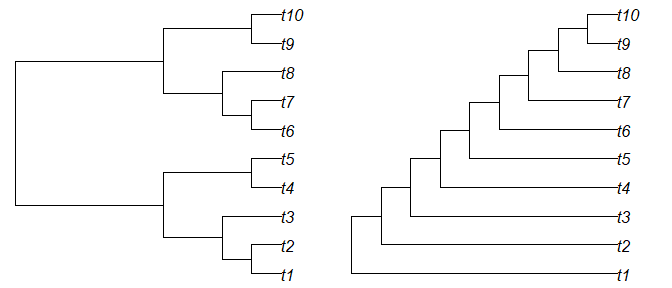# Using TBRDist

#### 2020-09-17

‘TBRDist’ is an R package to compare unrooted phylogenetic trees using the SPR, TBR and Replug distances.

## Loading trees

This document assumes familiarity with the process of loading trees into R.

We’ll work with some simple trees generated using the ‘TreeTools’ package.

library('TreeTools', quietly = TRUE, warn.conflicts = FALSE)

tree1 <- BalancedTree(10)
tree2 <- PectinateTree(10)

origPar <- par(mfrow = 1:2, mar = rep(0.2, 4)) # Set up plotting area
plot(tree1)
plot(tree2)## Calculating distances

After installing TBRDist (install.packages('TBRDist')), load the package into R with

library('TBRDist')

To generate an approximate SPR distance between two unrooted trees, use:

USPRDist(tree1, tree2)
##  2

For Replug distances, it’s:

ReplugDist(tree1, tree2)
##  2

For TBR distances, use:

TBRDist(tree1, tree2, exact = TRUE)
##  1

When calculating an exact TBR distance, we receive information on the maximum agreement forest for free (i.e. with no extra processing cost):

TBRDist(tree1, tree2, exact = TRUE, maf = TRUE)
## $tbr_exact ##  1 ## ##$maf_1
##  "(t4,t5,((t1,t2),t3)); ((t9,t10),t8,(t7,t6));"
##
## $maf_2 ##  "(((t1,t2),t3),t4,t5); ((t6,t7),(t9,t10),t8);" Once trees have more than about a dozen tips, it becomes slow to calculate the exact distance. In the interests of speed, we may wish to approximate the value of the TBR distance: TBRDist(tree1, tree2, exact = FALSE) ##$tbr_min
##  1
##
## $tbr_max ##  3 ## Comparing multiple trees To avoid multiple calls, each function can also be used to compare lists of trees (or multiPhylo objects) against a single tree: TBRDist(tree1, list(tree1, tree2), exact = TRUE, maf = TRUE) ##$tbr_exact
##  0 1
##
## $maf_1 ##  "((((t1,t2),t3),(t4,t5)),(t9,t10),((t6,t7),t8));" ##  "(t4,t5,((t1,t2),t3)); ((t9,t10),t8,(t7,t6));" ## ##$maf_2
##  "((((t1,t2),t3),(t4,t5)),(t9,t10),((t6,t7),t8));"
##  "(((t1,t2),t3),t4,t5); ((t6,t7),(t9,t10),t8);"

Or against each corresponding entry in a second list:

USPRDist(list(tree1, tree2, tree2), list(tree2, tree1, tree2))
##  2 2 0

## Citation

If you use ‘TBRDist’ in your research, please cite:

• Chris Whidden and Frederick A. Matsen IV (2017). Calculating the Unrooted Subtree-Prune-and-Regraft Distance. eprint arXiv:1511.07529.

Optionally, a citation to the ‘TBRDist’ R package would be welcome: